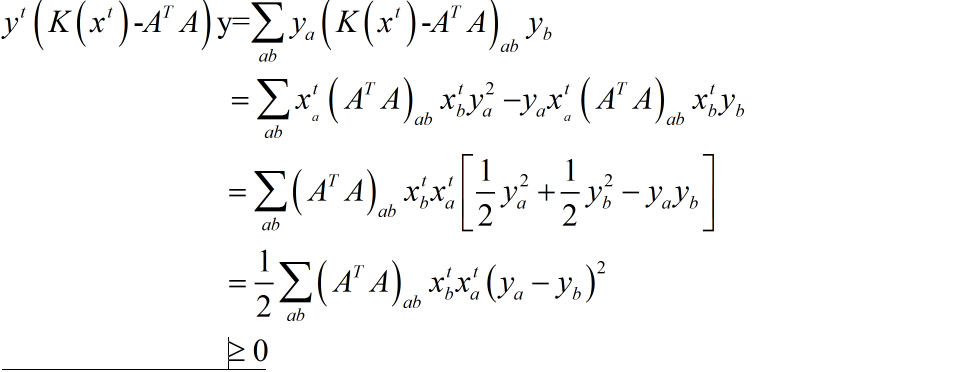## 奇异值分解

### 奇异值分解与Latent semantic indexing (LSI)

$M \approx {\rm{ }}{U^{\left( k \right)}}{\Sigma ^{\left( k \right)}}{V^{\left( k \right)}}^T]$

，其中$U$我们称为潜在语义或者是topic。这样我们在计算两个文档$M_i$和$M_j$的相似度的时候我们可以把两个文档在矩阵$U$上作投影然后再计算相似度，即

$\left. {\left\langle {{M_i}^TU,{M_j}^TU} \right.} \right\rangle$

1. “主题”是单位正交的

1. 主题中存在负的数值

But then when we compute similarly, two documents are
judged to be more similar based on a topic that they are both decidedly not about.This is another counter intuitive and undesirable property


## 非负矩阵分解##

##非负矩阵的求解##

###乘法更新求解NMF### 在乘法更新中目标函数的表达式为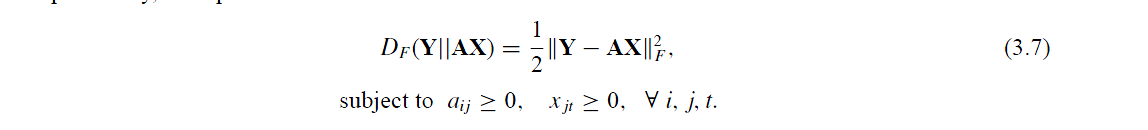然后我们对这个目标函数表达式求解KKT条件得到如下：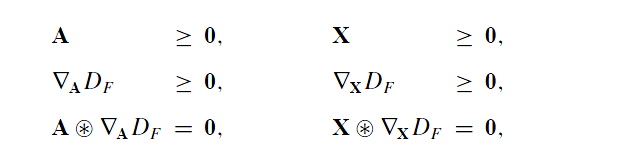其中梯度的表达式为：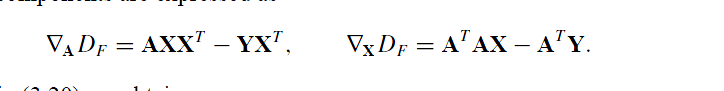结合上述的两组式子我们得到：即更新的规则为: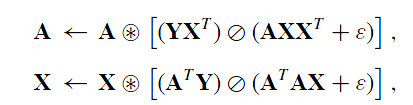###最小二乘求解NMF###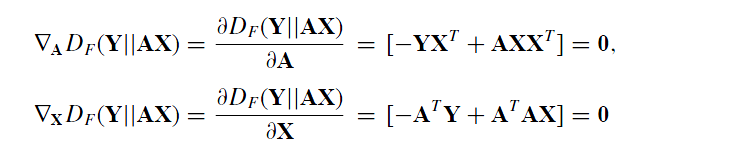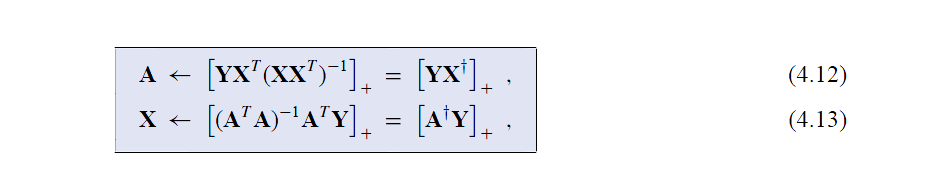###非负矩阵收敛证明###

####定义辅助函数####

1. $G\left( {x,x’} \right) \ge F\left( h \right)$
2. $G\left( {x,x} \right) = F\left( x \right)$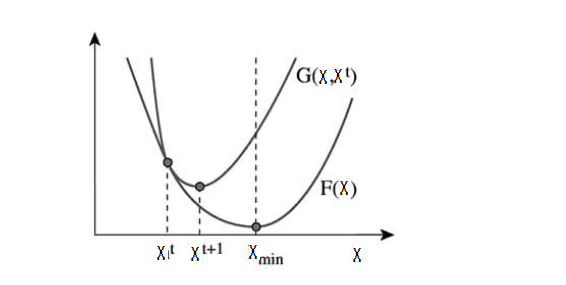$F\left( {{x^{t + 1}}} \right) = G\left( {{x^{t + 1}},{x^t}} \right) = G\left( {{x^t},{x^t}} \right) = F\left( {{x^t}} \right)$

####构造辅助函数#### 下面我们根据上面的性质对目标函数$\left|| {Y - AX} \right||$构造辅助函数G。 函数$F\left( x \right) = \frac{1}{2}\sum \limits_i {{{\left( {{y_i} - \sum\limits_a {{A_{ia}}{x_a}} } \right)}^2}}$的辅助函数$G\left( {{x},{x^t}} \right)$定义为：

$G\left( {x,{x^t}} \right) = F\left( {{x^t}} \right) + {\left( {x - {x^t}} \right)^T}\nabla F\left( {{x^t}} \right) + \frac{1}{2}{\left( {x - {x^t}} \right)^T}K\left( {{x^t}} \right)\left( {x - {x^t}} \right)$

${K_{ab}}\left( \right) = {\delta_{ab}}{\left( {{A^T}A{x^t}} \right)_a}/x_a^t$